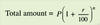# Compound Interest Flashcards Preview

## Maths in Practical Situation II > Compound Interest > Flashcards

Flashcards in Compound Interest Deck (9):
1

2

## What is the formula for calculating total amount using compound interest

### P = Principalr = compound interest per yearn = number of years3

## To calculate the compound interest, what must we do?

### We subtract the Principal from the Total amount.4

## Calculate the Total amount fromPrincipal = \$20000Compound interest rate = 2%No of years = 35

6

## Calculate the Total amount fromPrincipal = \$25000Compound interest rate = 2.5%No of years = 47

8

## Calculate the Total amount fromPrincipal = \$30000Compound interest rate = 1.5%No of years = 109